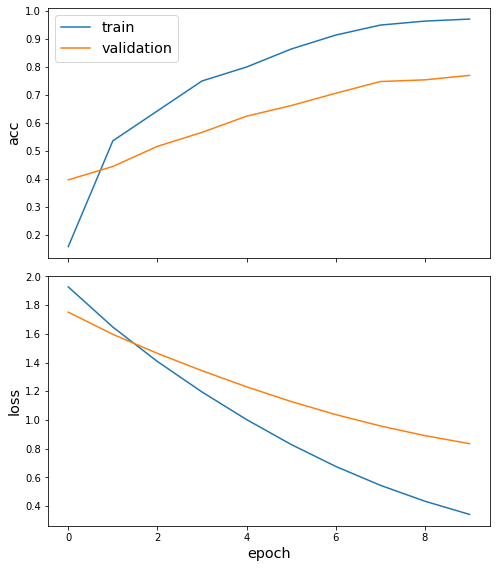# Interpreting nodes and edges with saliency maps in GAT¶

This demo shows how to use integrated gradients in graph attention networks to obtain accurate importance estimations for both the nodes and edges. The notebook consists of three parts:

setting up the node classification problem for Cora citation network training and evaluating a GAT model for node classification calculating node and edge importances for model’s predictions of query (“target”) nodes.

:

import networkx as nx
import pandas as pd
import numpy as np
from scipy import stats
import os
import time
import sys
import stellargraph as sg
from copy import deepcopy

from stellargraph.mapper import FullBatchNodeGenerator
from stellargraph.layer import GAT, GraphAttention

from tensorflow.keras import layers, optimizers, losses, metrics, models, Model
from sklearn import preprocessing, feature_extraction, model_selection
from tensorflow.keras import backend as K
import matplotlib.pyplot as plt
from stellargraph import datasets
from IPython.display import display, HTML
%matplotlib inline


:

dataset = datasets.Cora()
display(HTML(dataset.description))

The Cora dataset consists of 2708 scientific publications classified into one of seven classes. The citation network consists of 5429 links. Each publication in the dataset is described by a 0/1-valued word vector indicating the absence/presence of the corresponding word from the dictionary. The dictionary consists of 1433 unique words.
:

print(G.info())

StellarGraph: Undirected multigraph
Nodes: 2708, Edges: 5429

Node types:
paper: 
Features: float32 vector, length 1433
Edge types: paper-cites->paper

Edge types:
paper-cites->paper: 


### Splitting the data¶

For machine learning we want to take a subset of the nodes for training, and use the rest for validation and testing. We’ll use scikit-learn again to do this.

Here we’re taking 140 node labels for training, 500 for validation, and the rest for testing.

:

train_subjects, test_subjects = model_selection.train_test_split(
subjects, train_size=140, test_size=None, stratify=subjects
)
val_subjects, test_subjects = model_selection.train_test_split(
test_subjects, train_size=500, test_size=None, stratify=test_subjects
)

:

from collections import Counter

Counter(train_subjects)

:

Counter({'Theory': 18,
'Neural_Networks': 42,
'Genetic_Algorithms': 22,
'Case_Based': 16,
'Rule_Learning': 9,
'Probabilistic_Methods': 22,
'Reinforcement_Learning': 11})


### Converting to numeric arrays¶

For our categorical target, we will use one-hot vectors that will be fed into a soft-max Keras layer during training. To do this conversion …

:

target_encoding = preprocessing.LabelBinarizer()

train_targets = target_encoding.fit_transform(train_subjects)
val_targets = target_encoding.transform(val_subjects)
test_targets = target_encoding.transform(test_subjects)

all_targets = target_encoding.transform(subjects)


## Creating the GAT model in Keras¶

To feed data from the graph to the Keras model we need a generator. Since GAT is a full-batch model, we use the FullBatchNodeGenerator class to feed node features and graph adjacency matrix to the model.

:

generator = FullBatchNodeGenerator(G, method="gat", sparse=False)


For training we map only the training nodes returned from our splitter and the target values.

:

train_gen = generator.flow(train_subjects.index, train_targets)


Now we can specify our machine learning model, we need a few more parameters for this:

• the layer_sizes is a list of hidden feature sizes of each layer in the model. In this example we use two GAT layers with 8-dimensional hidden node features at each layer.

• attn_heads is the number of attention heads in all but the last GAT layer in the model

• activations is a list of activations applied to each layer’s output

• Arguments such as bias, in_dropout, attn_dropout are internal parameters of the model, execute ?GAT for details.

To follow the GAT model architecture used for Cora dataset in the original paper [Graph Attention Networks. P. Veličković et al. ICLR 2018 https://arxiv.org/abs/1803.07294], let’s build a 2-layer GAT model, with the second layer being the classifier that predicts paper subject: it thus should have the output size of train_targets.shape (7 subjects) and a softmax activation.

:

gat = GAT(
layer_sizes=[8, train_targets.shape],
generator=generator,
bias=True,
in_dropout=0,
attn_dropout=0,
activations=["elu", "softmax"],
normalize=None,
saliency_map_support=True,
)

:

# Expose the input and output tensors of the GAT model for node prediction, via GAT.in_out_tensors() method:
x_inp, predictions = gat.in_out_tensors()


### Training the model¶

Now let’s create the actual Keras model with the input tensors x_inp and output tensors being the predictions predictions from the final dense layer

:

model = Model(inputs=x_inp, outputs=predictions)
model.compile(
loss=losses.categorical_crossentropy,
weighted_metrics=["acc"],
)


Train the model, keeping track of its loss and accuracy on the training set, and its generalisation performance on the validation set (we need to create another generator over the validation data for this)

:

val_gen = generator.flow(val_subjects.index, val_targets)


Train the model

:

N = G.number_of_nodes()
history = model.fit(
train_gen, validation_data=val_gen, shuffle=False, epochs=10, verbose=2
)

Epoch 1/10
1/1 - 9s - loss: 1.9274 - acc: 0.1571 - val_loss: 1.7515 - val_acc: 0.3960
Epoch 2/10
1/1 - 2s - loss: 1.6477 - acc: 0.5357 - val_loss: 1.5972 - val_acc: 0.4440
Epoch 3/10
1/1 - 2s - loss: 1.4080 - acc: 0.6429 - val_loss: 1.4644 - val_acc: 0.5160
Epoch 4/10
1/1 - 2s - loss: 1.1955 - acc: 0.7500 - val_loss: 1.3436 - val_acc: 0.5660
Epoch 5/10
1/1 - 2s - loss: 1.0033 - acc: 0.8000 - val_loss: 1.2316 - val_acc: 0.6240
Epoch 6/10
1/1 - 2s - loss: 0.8298 - acc: 0.8643 - val_loss: 1.1291 - val_acc: 0.6620
Epoch 7/10
1/1 - 2s - loss: 0.6770 - acc: 0.9143 - val_loss: 1.0381 - val_acc: 0.7060
Epoch 8/10
1/1 - 2s - loss: 0.5456 - acc: 0.9500 - val_loss: 0.9591 - val_acc: 0.7480
Epoch 9/10
1/1 - 2s - loss: 0.4348 - acc: 0.9643 - val_loss: 0.8916 - val_acc: 0.7540
Epoch 10/10
1/1 - 2s - loss: 0.3428 - acc: 0.9714 - val_loss: 0.8356 - val_acc: 0.7700

:

sg.utils.plot_history(history)Evaluate the trained model on the test set

:

test_gen = generator.flow(test_subjects.index, test_targets)

test_metrics = model.evaluate(test_gen)
print("\nTest Set Metrics:")
for name, val in zip(model.metrics_names, test_metrics):
print("\t{}: {:0.4f}".format(name, val))


Test Set Metrics:
loss: 0.7781
acc: 0.7955


Check serialization

:

# Save model
model_json = model.to_json()
model_weights = model.get_weights()

:

# Load model from json & set all weights
model2 = models.model_from_json(model_json, custom_objects=sg.custom_keras_layers)
model2.set_weights(model_weights)
model2_weights = model2.get_weights()

:

pred2 = model2.predict(test_gen)
pred1 = model.predict(test_gen)
print(np.allclose(pred1, pred2))

True